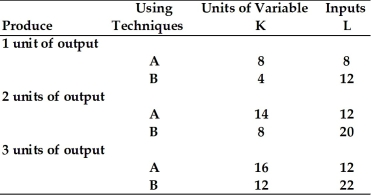# [Solved] Refer to the Information Provided in Table 8

Question 28
Multiple Choice

## Refer to the information provided in Table 8.1 below to answer the question(s) that follow.Table 8.1-Refer to Table 8.1. Assuming the price of labor (L) is \$5 per unit and the price of capital (K) is \$10 per unit, what production technique should this firm use to produce 2 units of output?

A) production technique A
B) production technique B
C) The firm is indifferent between production technique A and production technique B.
D) It is impossible to determine if the firm should select production technique A or B because total fixed costs are not given.

10+ million students use Quizplus to study and prepare for their homework, quizzes and exams through 20m+ questions in 300k quizzes.

Explore our library and get Economics Homework Help with various study sets and a huge amount of quizzes and questions

3.5K

Study sets

66.5K

Quizzes

5.9M

Questions

Upload material to get free accessInvite a friend and get free accessSubscribe and get an instant access